# Caffe中的优化方法

Solver的流程：

1.     设计好需要优化的对象，以及用于学习的训练网络和用于评估的测试网络。

2.     通过forward和backward迭代的进行优化来跟新参数

3.     定期的评价测试网络

4.     在优化过程中显示模型和solver的状态

每一步迭代的过程

1.     通过forward计算网络的输出和loss

2.     通过backward计算网络的梯度

3.     根据solver方法，利用梯度来对参数进行更新

4.     根据learning rate，history和method来更新solver的状态

## 1. Methods

Solver方法一般用来解决loss函数的最小化问题。对于一个数据集D，需要优化的目标函数是整个数据集中所有数据loss的平均值。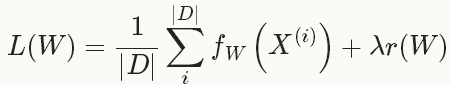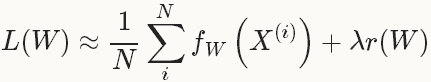### 1.1 SGD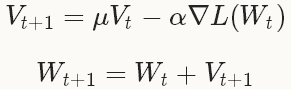base_lr: 0.01     # begin training at a learning rate of0.01 = 1e-2 lr_policy: "step" # learning ratepolicy: drop the learning rate in "steps"                  # by a factor of gamma everystepsize iterations gamma: 0.1        # drop the learning rate by a factor of10                  # (i.e., multiply it by afactor of gamma = 0.1) stepsize: 100000  # drop the learning rate every 100K iterations max_iter: 350000  # train for 350K iterations total momentum: 0.9

Note that the momentum setting μ effectively multiplies the size of your updates by a factor of 11−μ after many iterations of training, so if you increase μ, it may be a good idea to decrease α accordingly (and vice versa).

For example, with μ=0.9, we have an effective update size multiplier of 11−0.9=10. If we increased the momentum to μ=0.99, we’ve increased our update size multiplier to 100, so we should drop α (base_lr) by a factor of 10.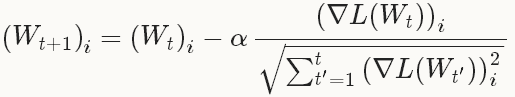### 1.3 NAG

Nesterov 的加速梯度法（Nesterov’s accelerated gradient）作为凸优化中最理想的方法，其收敛速度可以达到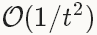而不是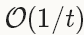。但由于深度学习中的优化问题往往是非平滑的以及非凸的（non-smoothness and non-convexity），在实践中NAG对于某类深度学习的结构可以成为非常有效的优化方法，比如deep MNIST autoencoders。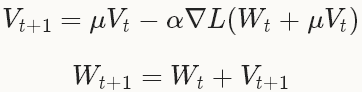## 2. 参考：

 L. Bottou. Stochastic Gradient Descent Tricks. Neural Networks: Tricks of the Trade: Springer, 2012.
 A. Krizhevsky, I. Sutskever, and G. Hinton. ImageNet Classification with Deep Convolutional Neural Networks. Advances in Neural Information Processing Systems, 2012.
 J. Duchi, E. Hazan, and Y. Singer. Adaptive Subgradient Methods for Online Learning and Stochastic Optimization. The Journal of Machine Learning Research, 2011.
 Y. Nesterov. A Method of Solving a Convex Programming Problem with Convergence Rate O(1/k√). Soviet Mathematics Doklady, 1983.
 I. Sutskever, J. Martens, G. Dahl, and G. Hinton. On the Importance of Initialization and Momentum in Deep Learning. Proceedings of the 30th International Conference on Machine Learning, 2013.
 http://caffe.berkeleyvision.org/tutorial/solver.html

©️2019 CSDN 皮肤主题: 技术黑板 设计师: CSDN官方博客